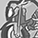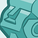Announcements
Product Release Webinar: Qlik Insider airing December 6! REGISTER TODAY!
cancel
Showing results for
Did you mean:Contributor III

## Display calculated dimension in Chart title

I have a chart dimension that returns just 1 value - by design.

=if(aggr(rank(sum(Cost),4),H_Process) = 2, H_Process)

How can I display the value/output of this expression in the chart title?

Thanks

Labels (2)

• ### Layout & Visualizations

1 Solution

Accepted SolutionsContributor III
Author

Thank you for the help.  I didn't know where to start.

I found

Concat(
distinct
{<H_Process= {"=aggr(rank(sum(Cost),4),H_Process) = 2"}>}
H_Process
)

did the trick.

2 RepliesCreator II
``````Concat(
distinct
{<H_Process= {"=Rank(sum(Cost))=2 "}>}
H_Process
)``````Contributor III
Author

Thank you for the help.  I didn't know where to start.

I found

Concat(
distinct
{<H_Process= {"=aggr(rank(sum(Cost),4),H_Process) = 2"}>}
H_Process
)

did the trick.Community Browser